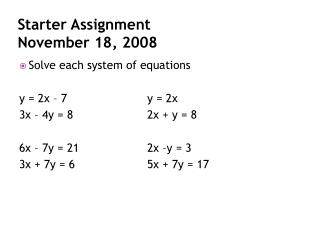DownloadDownload PresentationStarter Assignment November 18, 2008

# Starter Assignment November 18, 2008

Télécharger la présentation## Starter Assignment November 18, 2008

- - - - - - - - - - - - - - - - - - - - - - - - - - - E N D - - - - - - - - - - - - - - - - - - - - - - - - - - -
##### Presentation Transcript

1. Starter Assignment November 18, 2008 • Solve each system of equations y = 2x – 7 y = 2x 3x – 4y = 8 2x + y = 8 6x – 7y = 21 2x –y = 3 3x + 7y = 6 5x + 7y = 17

2. UNIt 7 Law of Exponents • Objective • Students will simplify expressions involving exponents. • Standard • Agenda • Starter Assignment, Homework Check and Review, Multiplying Monomials, Homework Assignment

3. Unit 6 Exponents and Polynomials Laws of Exponents

4. Objectives • Multiply monomials. • Divide monomials. • Express numbers in scientific notation and standard notation. • Add polynomials • Subtract polynomials. • Multiply polynomials. (FOIL Method)

5. What is a monomial? • A monomial is a number, a variable, or a product of a number and one or more variables. • Examples of monomials • -5, 10, 307, -250 (Also called constants) • a, b, x, y • 2x, -3y, 7ab, -12xyz

6. Exponents • The exponent indicates how many times the base is used a factor. • 53 • 42 • -16 • a5

7. How we use exponents in real life? • We use exponents to write really big numbers as more manageable numbers. • Bankers use exponents to determine how much interest we have on our savings accounts. • Doctors use exponents to see how fast a colony of bacteria grow. • In Geometry, exponents are used to calculate area and volume.

8. Three Key Concepts • Product of Powers • Power of a Power • Power of a Product

9. Product of Powers • To multiply two powers that have the same base, add the exponents. xm• xn = xm + n

10. Complete the table

11. Practice Problems • 52• 53 • a4 • a2

12. Practice Problems • (6x3)(4x3) • (5t)(-30t2)

13. Practice Problems • (3x2)(50xy2) • (-2c2)(3c3)

14. Connection: Geometry • The volume of a right rectangular prism can be found by using the formula V= lwh, where l is the length, w is the width, and h is the height. Suppose that a prism has a length of 2xy, a width of 3xy, and a height of 6xyz. Find the volume.

15. Power-of-a-Power • To find the power of a power, multiply the exponents. • (xm)n = x m•n

16. Complete the Table

17. Practice Problems • (p2)5 • (r4)2

18. Practice Problems • (23)4 • (103)2

19. Practice Problems • (103)4 • [(x4)2]3

20. Power of a Product • To find the power of a product, find the power of each factor and multiply. • (xy)m = xmym. • I like to call this property “exponent distribution”.

21. Practice Problems • (xy2)2 • (a3b)2

22. Practice Problems • (2 • 3)3 • (2 • 5)5

23. Practice Problems • (x2y3)5

24. Homework page 413 (21- 13 All problems) page 413 (21- 13 All problems)

25. Third Block • You need to stay to come in Thursday morning or afternoon to finish your test. • Darryl • Tanisha • Deon

26. Fourth Block • You need to stay to come in Thursday morning or afternoon to finish your test. • Taylor • Tyrean